Question

1. Certain standardized math exams have a mean of 100 and a standard deviation of 60. Of a sample of 36 students who take this exam, what percent could you expect to score between 100 and 120?

A)50

B)47.5

C)97.5

D)49.85

2.Certain standardized math exams have a mean of 100 and a standard deviation of 60. Of a sample of 36 students who take this exam, what percent could you expect to score above 120?

A)2.5

B)2.35

C)97.5

D)13.5

3. Certain standardized math exams have a mean of 100 and a standard deviation of 60. Of a sample of 36 students who take this exam, what percent could you expect to score above 70?

 A) 99.85 B) 95 C) 97.5 D) 0.15

4.Certain standardized math exams have a mean of 100 and a standard deviation of 60. Of a sample of 36 students who take this exam, what percent could you expect to score between 80 and 110?

A)84

B)81.5

C)83.85

D)85

5.Certain standardized math exams had a mean of 120 and a standard deviation of 20. Of students who take this exam, what percent could you expect to score below 100?

6. A mean could be a

A) Statistics only

B) Parameter only

C) Statistics and parameter

Solution :

Given that ,

mean =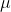= 100

standard deviation =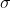= 60

1)

n = 36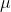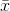= 100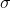=/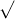n = 60 /36 = 60 / 6 = 10

P(100 << 120) = P((100 - 100) / 10<(-) /< (120 - 100) / 10))

= P(0 < Z < 2)

= P(Z < 2) - P(Z < 0)

= 0.9750 - 0.5

= 0.475

Percent = 47.5

2)

P(> 120) = 1 - P(< 120)

= 1 - P((-) /< (120 - 100) / 10)

= 1 - P(z < 2)

= 1 - 0.975

= 0.025

Percent = 2.5%

3)

P(< 70) = P((-) /< (70 - 100) / 10)

= P(z < -3)

= 0.9985

Percent = 99.85

4)

P(80 << 110) = P((80 - 100) / 10<(-) /< (110 - 100) / 10))

= P(-2 < Z < 1)

= P(Z < 1) - P(Z < -2)

= 0.84 - 0.025

= 0.815

Percent = 81.5

5)

P(< 100) = P((-) /< (100 - 100) / 10)

= P(z < 0)

= 0.50

Percent = 50%

6)

A mean could be a Statistics and parameter .

#### Earn Coins

Coins can be redeemed for fabulous gifts.

Similar Homework Help Questions
• ### Certain standardized math exams have a mean of 100 and a standard deviation of 60. Of a sample of 36 students who take this exam, what percent could you expect to score between 80 and 90?

Certain standardized math exams have a mean of 100 and a standard deviation of 60. Of a sample of 36 students who take this exam, what percent could you expect to score between 80 and 90?

• ### The mean SAT math score in 2007 was 525 with a standard deviation of 114. An...

The mean SAT math score in 2007 was 525 with a standard deviation of 114. An educational psychologist believes that students who take at least 4 years of English classes in High School score better on the SAT math exam. The psychologist obtains a random sample of 40 high school students who completed at least 4 years of high school English classes. The mean math exam score of these students was 540. Conduct an appropriate hypothesis test at the Alpha...

• ### (2 points) For students in a certain region, scores of students on a standardized test approximately...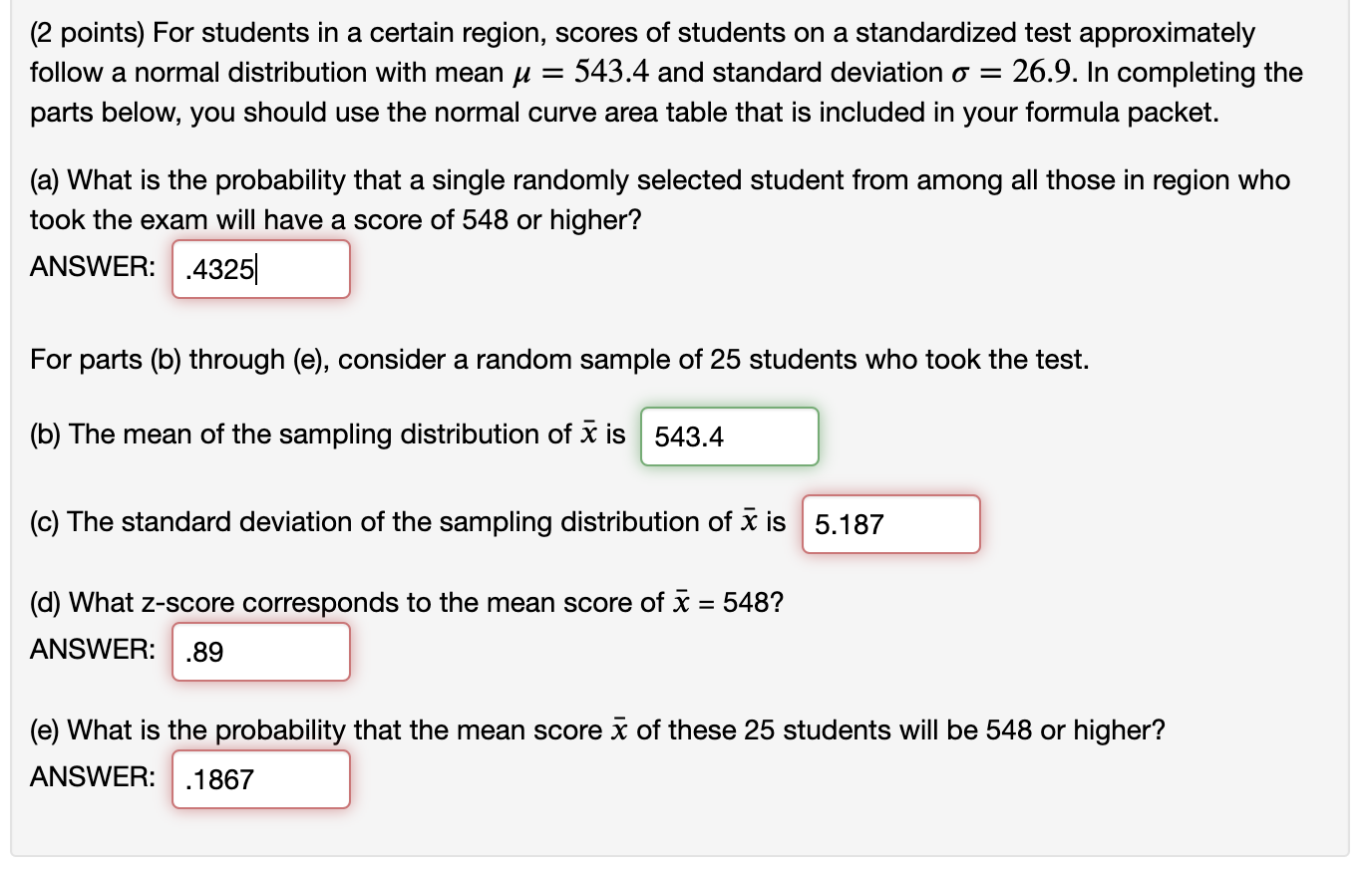(2 points) For students in a certain region, scores of students on a standardized test approximately follow a normal distribution with mean u = 543.4 and standard deviation o = 26.9. In completing the parts below, you should use the normal curve area table that is included in your formula packet. (a) What is the probability that a single randomly selected student from among all those in region who took the exam will have a score of 548 or higher?...

• ### Question 5 Estimating Mean SAT Math Score Type numbers in the boxes. 7 Part 1: 5...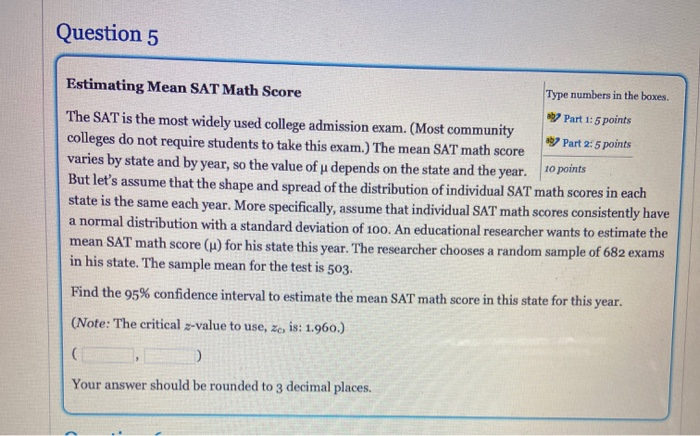Question 5 Estimating Mean SAT Math Score Type numbers in the boxes. 7 Part 1: 5 points The SAT is the most widely used college admission exam. (Most community Part 2:5 points colleges do not require students to take this exam.) The mean SAT math score varies by state and by year, so the value of u depends on the state and the year. to points But let's assume that the shape and spread of the distribution of individual SAT...

• ### Do students tend to improve their Math SAT scores the second time they take the test?...

Do students tend to improve their Math SAT scores the second time they take the test? We take a random sample of 100 hundred students who took the test twice. The mean score and the standard deviation of these 100 students on the first try are 500 and 90 respectively; the mean score and the standard deviation of these 100 students on the second try are 530 and 92 respectively. We also examine the change in Math SAT score (second...

• ### A standardized test's scores are normally distributed with a mean a 500 and a standard deviation...

A standardized test's scores are normally distributed with a mean a 500 and a standard deviation of 100. If 1200 students take the test, how many would you expect to score over 650? Round your answer to the nearest whole number.

• ### 1.) The scores for the final exam in Math 2250 is normally distributed with a mean...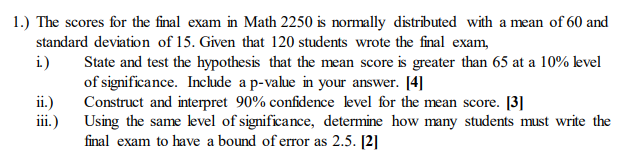1.) The scores for the final exam in Math 2250 is normally distributed with a mean of 60 and standard deviation of 15. Given that 120 students wrote the final exam, i.) State and test the hypothesis that the mean score is greater than 65 at a 10% level of significance. Include a p-value in your answer. 141 ii.) Construct and interpret 90% confidence level for the mean score.  Using the same level of significance, determine how many students...

• ### ROUND to 3 decimal places Type numbers in the boxes. Estimating Mean SAT Math Score ay...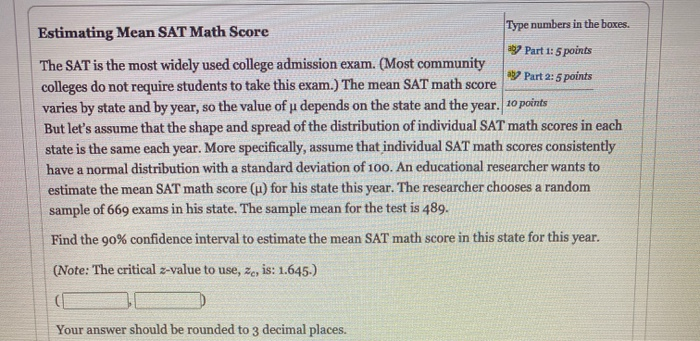ROUND to 3 decimal places Type numbers in the boxes. Estimating Mean SAT Math Score ay Part 1: 5 points The SAT is the most widely used college admission exam. (Most community ay Part 2: 5 points colleges do not require students to take this exam.) The mean SAT math score varies by state and by year, so the value of u depends on the state and the year. 10 points But let's assume that the shape and spread of...

• ### 7 In a certain year, when she was a high school senior, Idonna scored 679 on...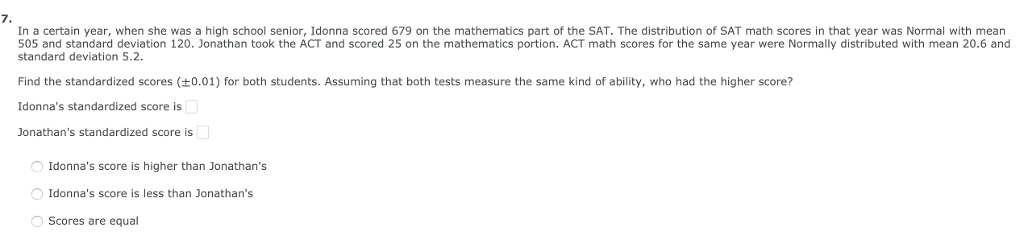7 In a certain year, when she was a high school senior, Idonna scored 679 on the mathematics part of the SAT. The distribution of SAT math scores in that year was Normal with mean 505 and standard deviation 120. Jonathan took the ACT and scored 25 on the mathematics portion. ACT math scores for the same year were Normally distributed with mean 20.6 and standard deviation 5.2 Find the standardized scores ±0.01) for both students. Assuming that both tests...

• ### Example: The time to complete a standardized exam is approximately normal with a mean of 70...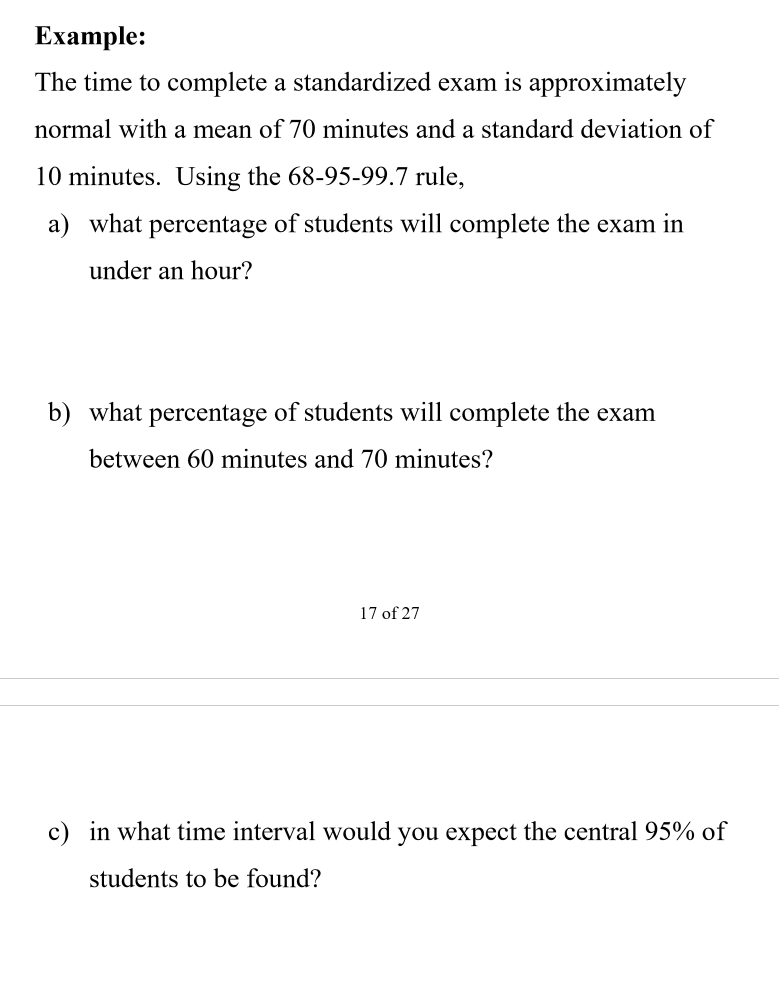Example: The time to complete a standardized exam is approximately normal with a mean of 70 minutes and a standard deviation of 10 minutes. Using the 68-95-99.7 rule, a) what percentage of students will complete the exam in under an hour? b) what percentage of students will complete the exam between 60 minutes and 70 minutes? 17 of 27 c) in what time interval would you expect the central 95% of students to be found?